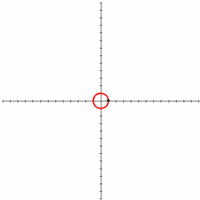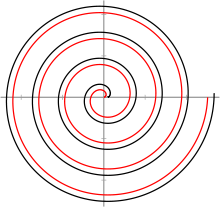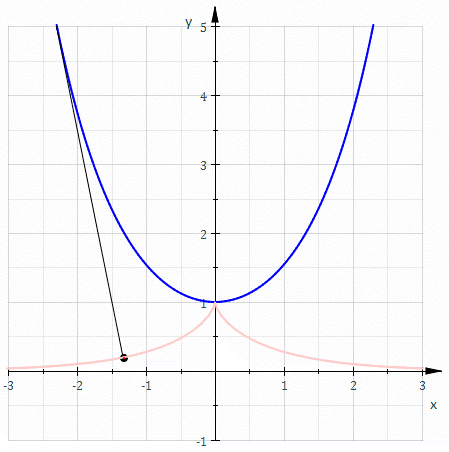# Involute

In the differential geometry of curves, an involute (also known as evolvent) is a curve obtained from another given curve by attaching an imaginary taut string to the given curve and tracing its free end as it is wound onto that given curve; or in reverse, unwound. It is a roulette wherein the rolling curve is a straight line containing the generating point. For example, an involute approximates the path followed by a tetherball as the connecting tether is wound around the center pole. If the center pole has a circular cross-section, then the curve is an involute of a circle.

Alternatively, another way to construct the involute of a curve is to replace the taut string by a line segment that is tangent to the curve on one end, while the other end traces out the involute. The length of the line segment is changed by an amount equal to the arc length traversed by the tangent point as it moves along the curve.

The evolute of an involute is the original curve, less portions of zero or undefined curvature. Compare Media:Evolute2.gif and Media:Involute.gif

If the functionis a natural parametrization of the curve (i.e.,for all s), then :parametrizes the involute.

The notions of the involute and evolute of a curve were introduced by Christiaan Huygens in his work titled Horologium oscillatorium sive de motu pendulorum ad horologia aptato demonstrationes geometricae (1673).

## Involute of a Parametrically Defined Curve

Equations of an involute curve for a parametrically defined functionare:## Examples

### Involute of a circleThe involute of a circle by
unwinding a stringThe involute of a circle (black) is not identical to the Archimedean spiral (red) in a trivial manner.

The involute of a circle resembles, but is not, an Archimedean spiral.

Its successive turns are parallel curves with constant separation distance, a property which is often (inaccurately) ascribed to the Archimedean spiral.whereis the radius of the circle, andis the angle in radians (). The counterclockwise spiral is made with positive values of, and the clockwise spiral is made with the negative values of.

• In polar coordinatesthe involute of a circle has the parametric equation:whereis the radius of the circle andis an angle parameter in radians () equal to(so).

With that parameterwithit can be written in the form:.

From the Cartesian coordinates and the first polar coordinates, can be seen that:so:#### Curve length

The arc length of the above curve foris#### Application

Leonhard Euler proposed to use the involute of the circle for the shape of the teeth of toothwheel gear, a design which is the prevailing one in current use, called involute gear.

### Involute of a catenaryThe involute of a catenary, a tractrix.

The involute of a catenary through its vertex is a tractrix. In Cartesian coordinates the curve follows:Where: t is a parameter and sech is the hyperbolic secant (1/cosh(t))

Derivative

Withwe haveand.

Substituteto get.

### Involute of a cycloid

One involute of a cycloid is a congruent cycloid. In cartesian coordinates the curve follows:Where t is the angle and r is the radius

## Application

The involute has some properties that makes it extremely important to the gear industry: If two intermeshed gears have teeth with the profile-shape of involutes (rather than, for example, a "classic" triangular shape), they form an involute gear system. Their relative rates of rotation are constant while the teeth are engaged, and also, the gears always make contact along a single steady line of force. With teeth of other shapes, the relative speeds and forces rise and fall as successive teeth engage, resulting in vibration, noise, and excessive wear. For this reason, nearly all modern gear teeth bear the involute shape.

The involute of a circle is also an important shape in gas compressing, as a scroll compressor can be built based on this shape. Scroll compressors make less sound than conventional compressors, and have proven to be quite efficient.